## 2.4 The Standard Model Representation

We are now in a position to put the entire Standard Model together in a single picture, much as we combined the isospinand hypercharge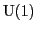into the electroweak gauge group,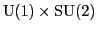, in Section 2.3.3. We then tensored the hyperchargerepresentations with the isospinrepresentations to get the electroweak representations.

Now let us take this process one step further, by bringing in a factor of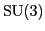, for the color symmetry, and tensoring the representations ofwith the representations of. Doing this, we get the Standard Model. The Standard Model has this gauge group: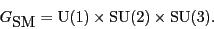The fundamental fermions described by the Standard Model combine to form representations of this group. We know what these are, and describe all of them in Table 1.

Table: Fundamental fermions as representations of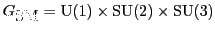The Standard Model Representation Name Symbol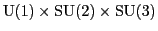rep Left-handed leptons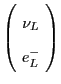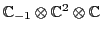Left-handed quarks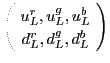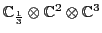Right-handed neutrino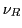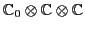Right-handed electron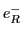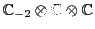Right-handed up quarks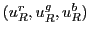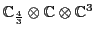Right-handed down quarks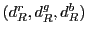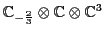All of the representations of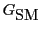in the left-hand column are irreducible, since they are made by tensoring irreps of this group's three factors,,and. This is a general fact: if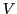is an irrep of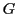, and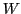is an irrep of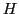, then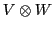is an irrep of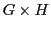. Moreover, all irreps ofarise in this way.

On the other hand, if we take the direct sum of all these irreps,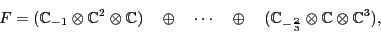we get a reducible representation containing all the first-generation fermions in the Standard Model. We call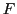the fermion representation. If we take the dual of, we get a representation describing all the antifermions in the first generation. And taking the direct sum of these spaces: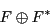we get a representation ofthat we will call the Standard Model representation. It contains all the first-generation elementary particles in the Standard Model. It does not contain the gauge bosons or the mysterious Higgs.

The fermions living in the Standard Model representation interact by exchanging gauge bosons that live in the complexified adjoint representation of. We have already met all of these, and we collect them in Table 2.

Table 2: Gauge bosons
 Gauge Bosons Force Gauge Boson Symbol Electromagnetism Photon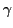Weak forceand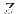bosons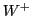,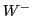andStrong force Gluons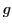Of all the particles and antiparticles in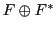, exactly two of them are fixed by the action of. These are the right-handed neutrino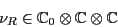and its antiparticle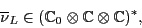both of which are trivial representations of; they thus do not participate in any forces mediated by the gauge bosons of the Standard Model. They might interact with the Higgs boson, but very little about right-handed neutrinos is known with certainty at this time.

2010-01-11